# RS Aggarwal Solutions for Class 9 Chapter 10: Quadrilaterals Exercise 10A

## RS Aggarwal Solutions for Class 9 Maths Exercise 10A PDF

RS Aggarwal solutions are an educational aid which helps students to learn easy and difficult problems. It consists of solutions which are prepared in an organized manner by subject experts at BYJU’S. The students can obtain the RS Aggarwal solutions for free in PDF format. This exercise is the introduction which explains types of quadrilaterals. The solutions help students to clear their doubts while solving problems and to prepare more efficiently for the board exams. RS Aggarwal Solutions for Class 9 Maths Chapter 10 Quadrilaterals Exercise 10A are provided here.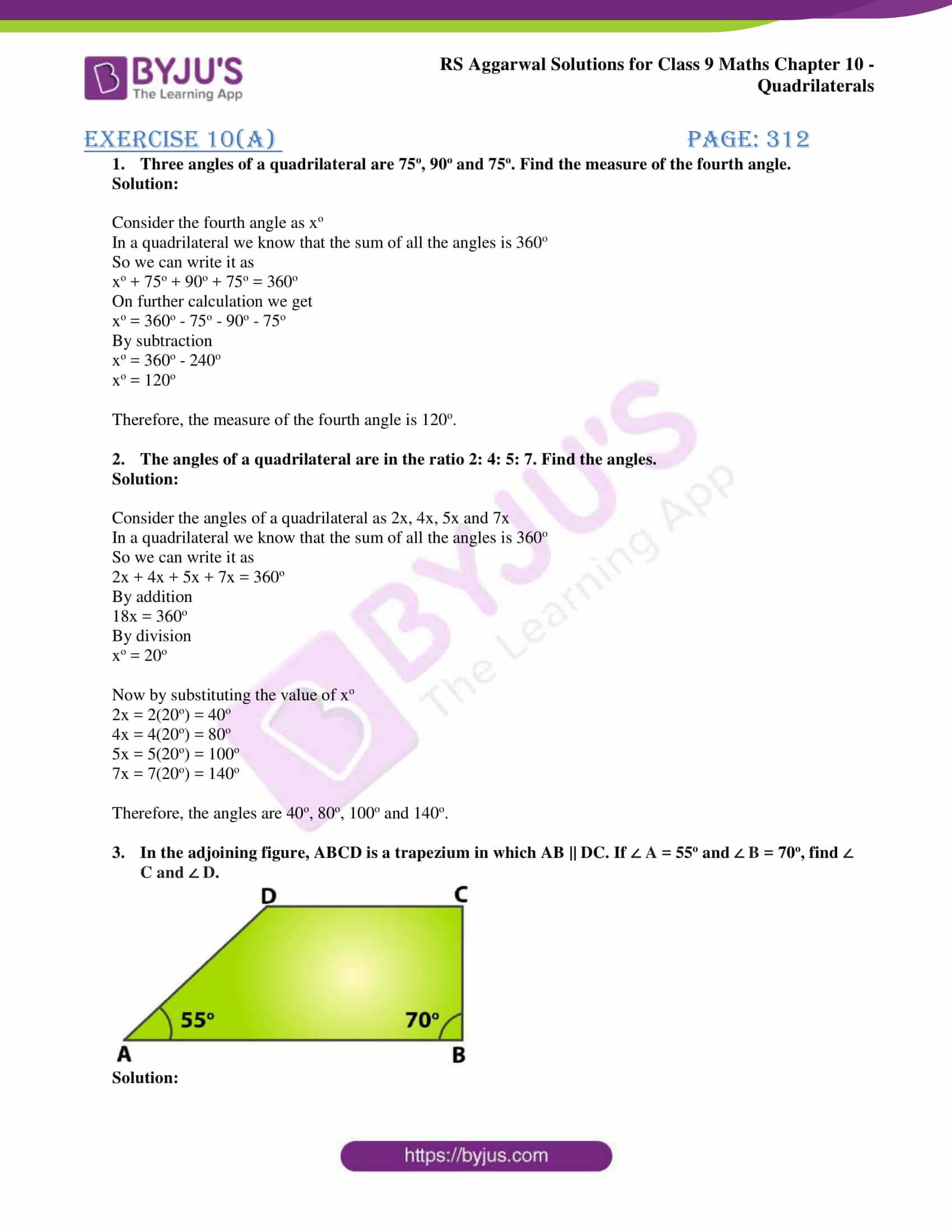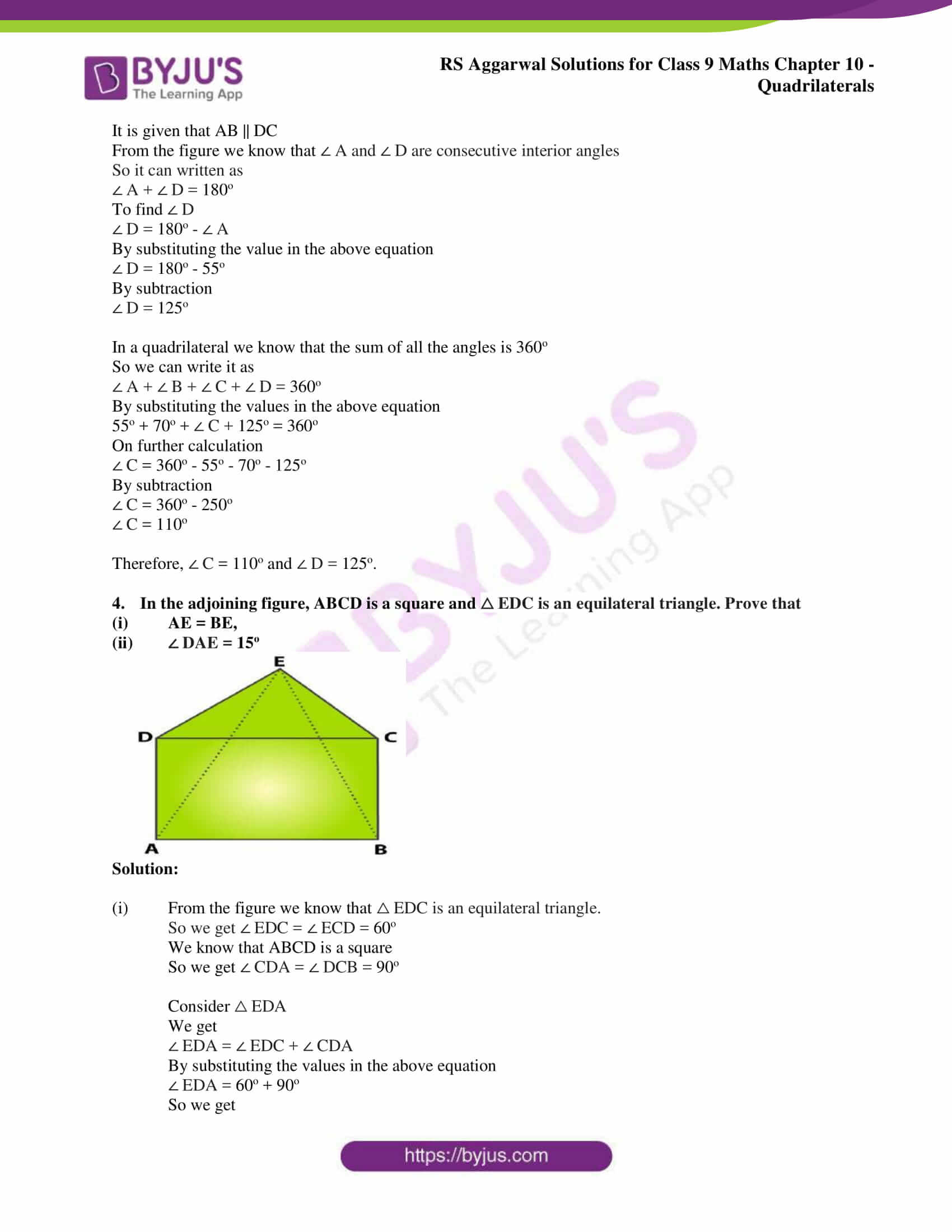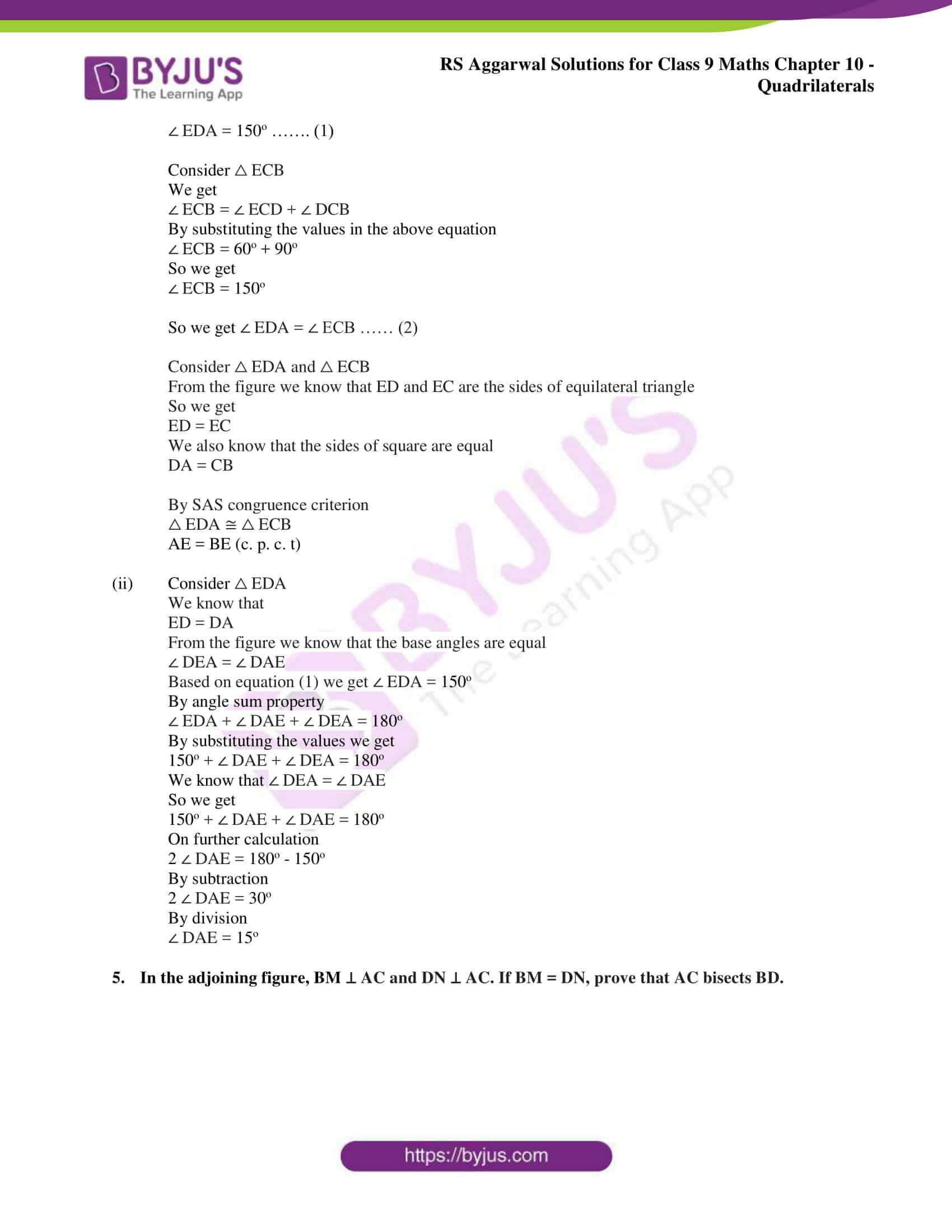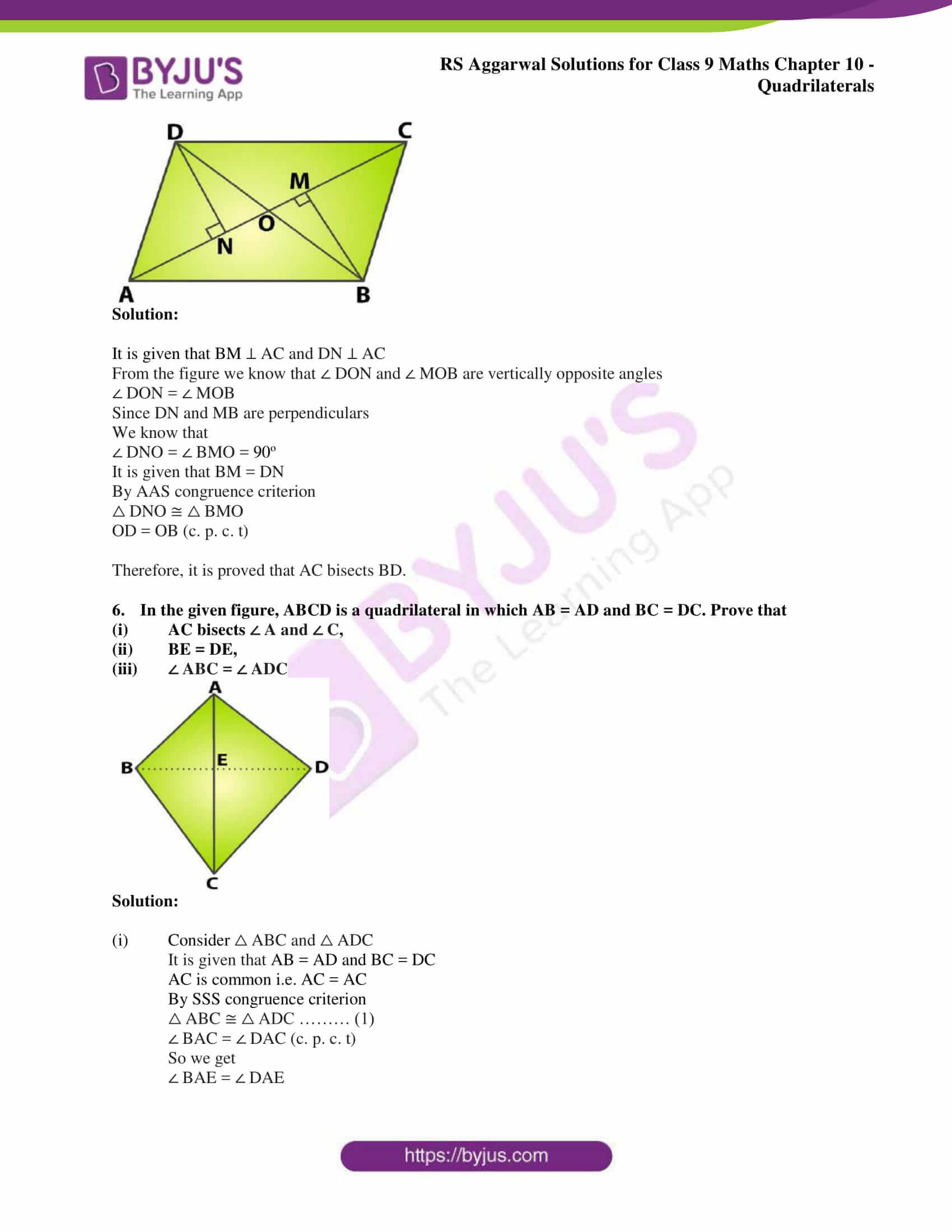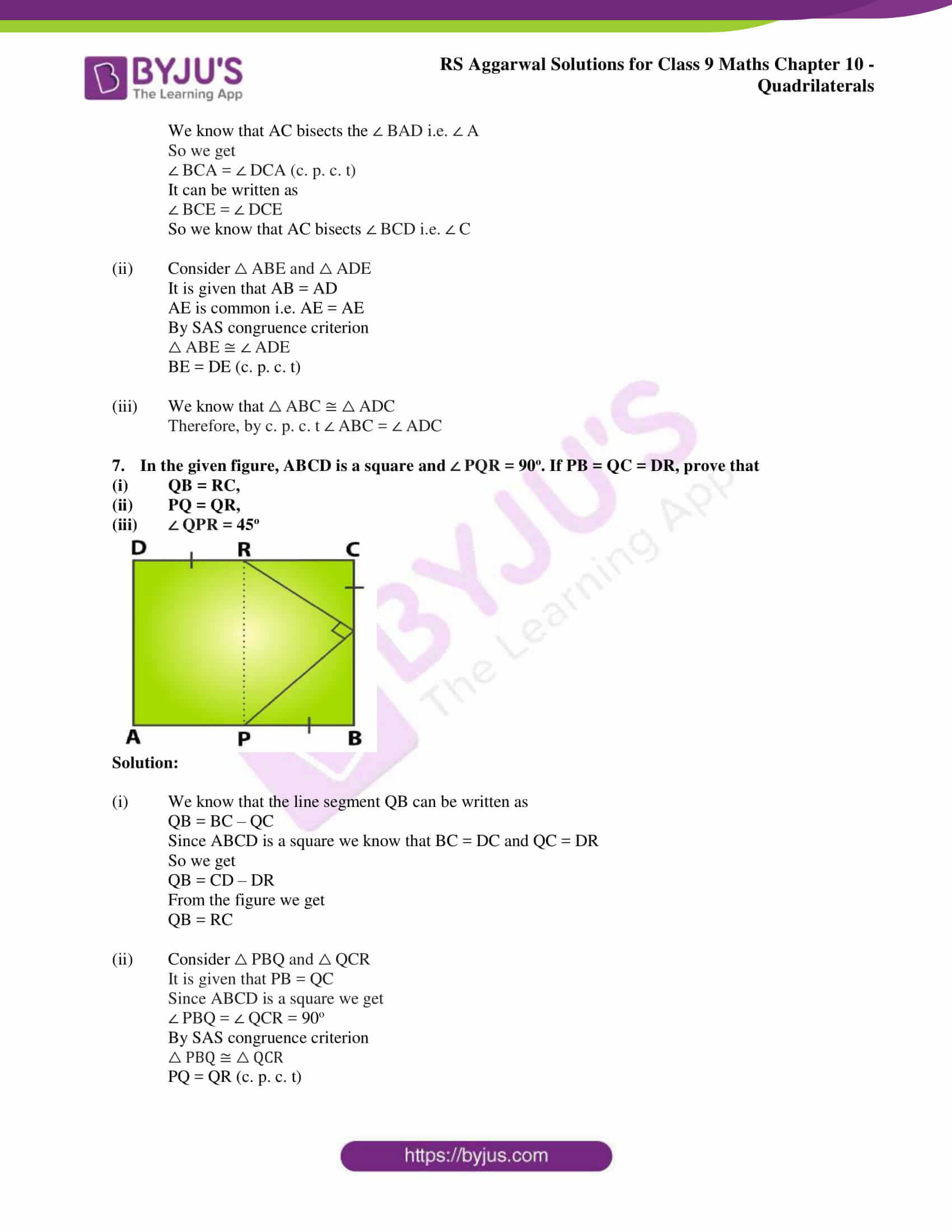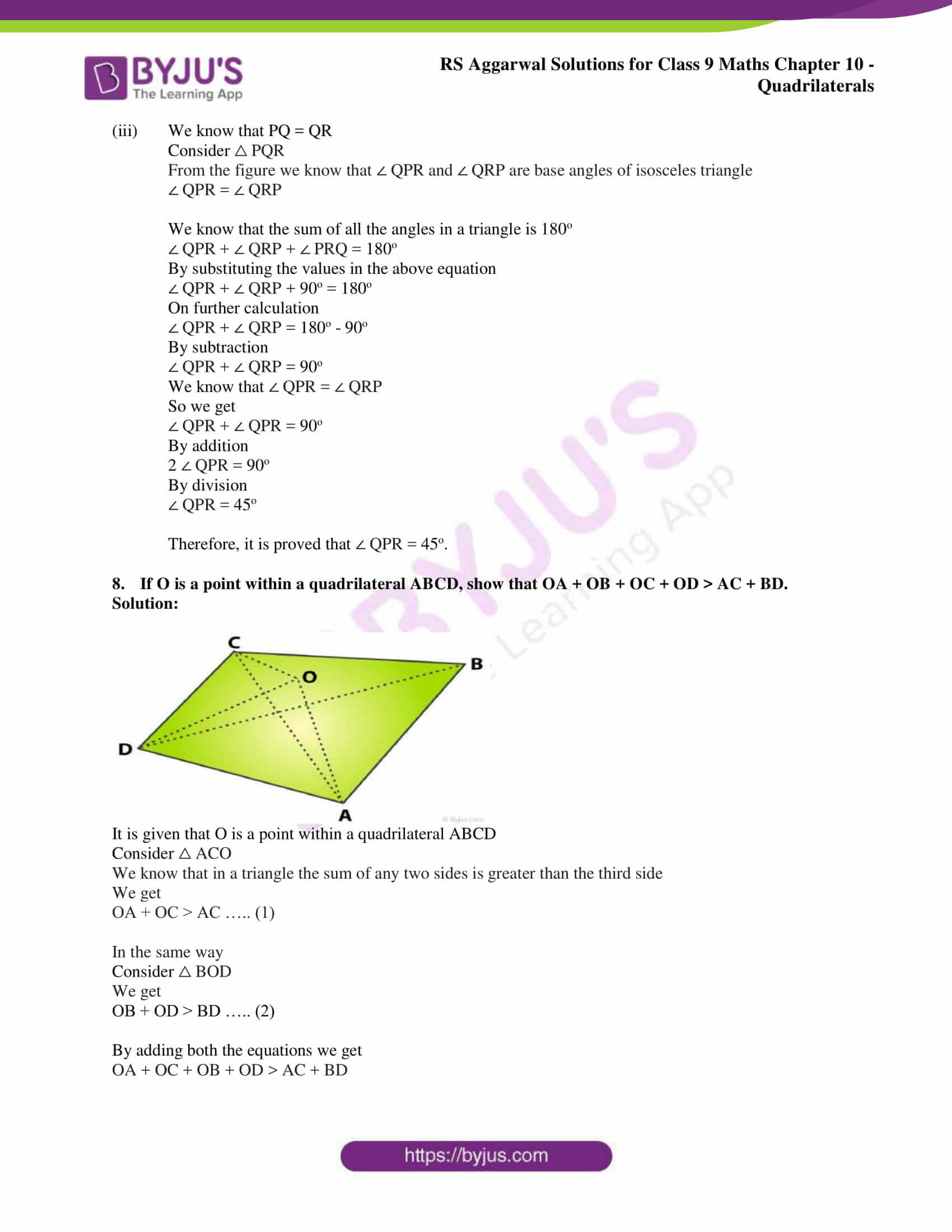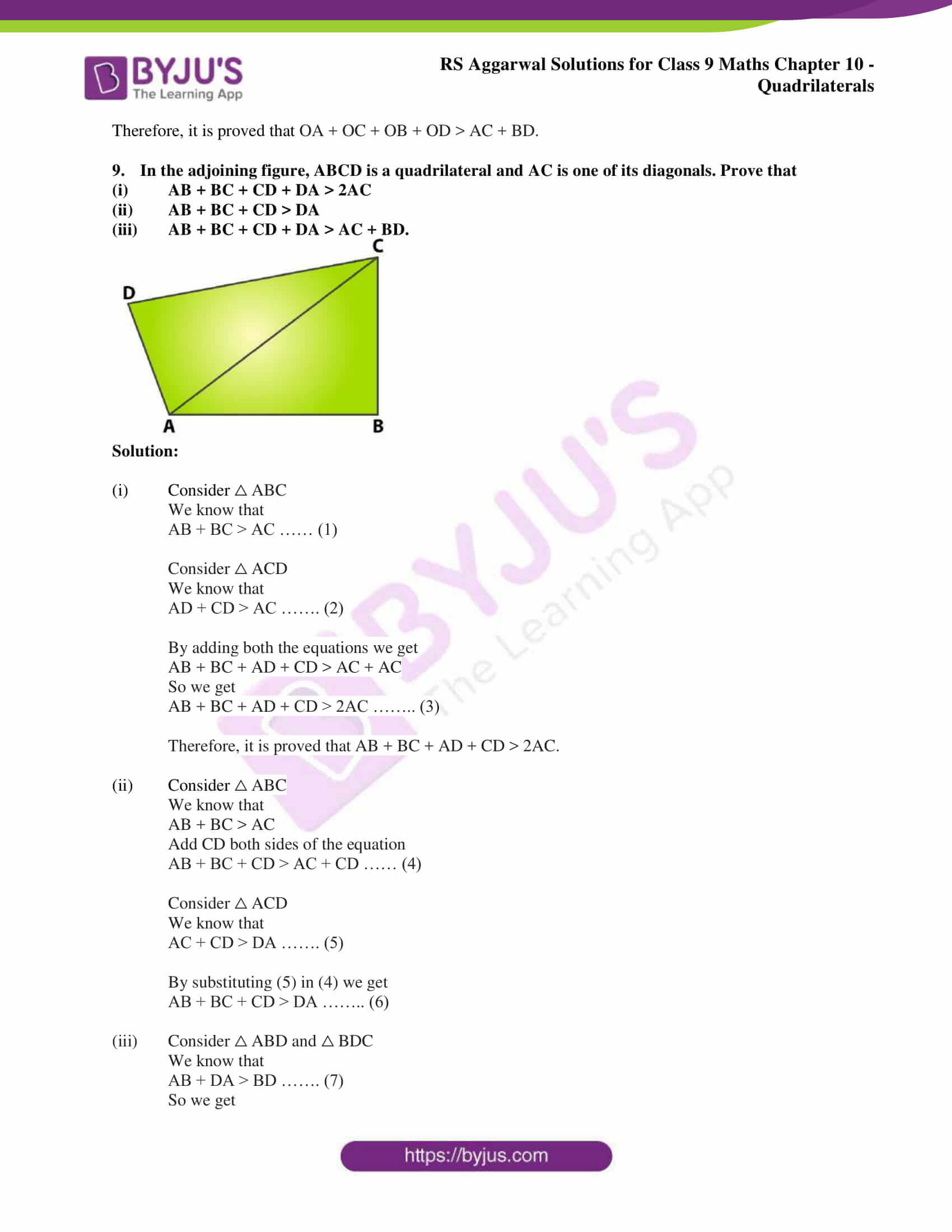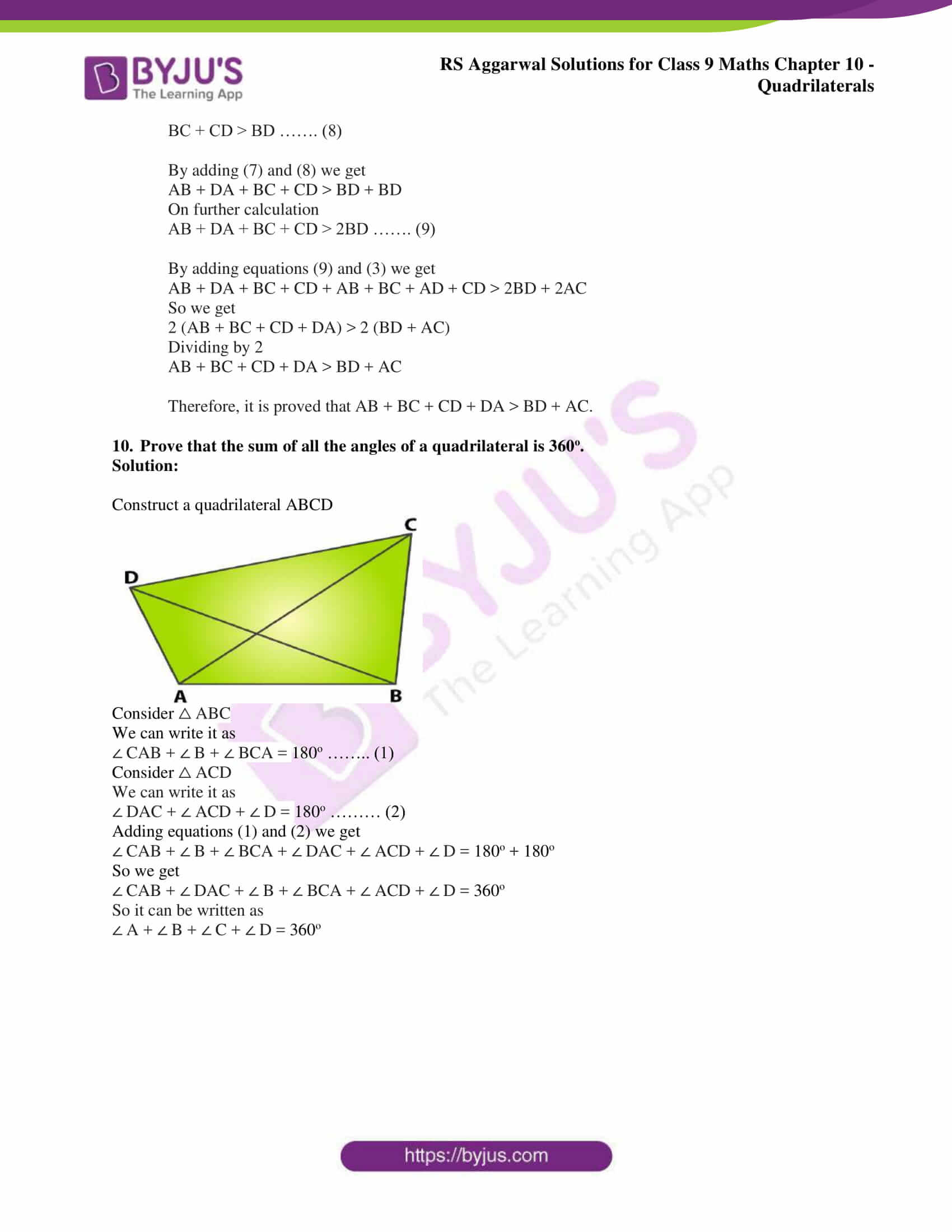## Access RS Aggarwal Solutions for Class 9 Chapter 10: Quadrilaterals Exercise 10A

1. Three angles of a quadrilateral are 75o, 90o and 75o. Find the measure of the fourth angle.

Solution:

Consider the fourth angle as xo

In a quadrilateral we know that the sum of all the angles is 360o

So we can write it as

xo + 75o + 90o + 75o = 360o

On further calculation we get

xo = 360o – 75o – 90o – 75o

By subtraction

xo = 360o – 240o

xo = 120o

Therefore, the measure of the fourth angle is 120o.

2. The angles of a quadrilateral are in the ratio 2: 4: 5: 7. Find the angles.

Solution:

Consider the angles of a quadrilateral as 2x, 4x, 5x and 7x

In a quadrilateral we know that the sum of all the angles is 360o

So we can write it as

2x + 4x + 5x + 7x = 360o

18x = 360o

By division

xo = 20o

Now by substituting the value of xo

2x = 2(20o) = 40o

4x = 4(20o) = 80o

5x = 5(20o) = 100o

7x = 7(20o) = 140o

Therefore, the angles are 40o, 80o, 100o and 140o.

3. In the adjoining figure, ABCD is a trapezium in which AB || DC. If ∠ A = 55o and ∠ B = 70o, find ∠ C and ∠ D.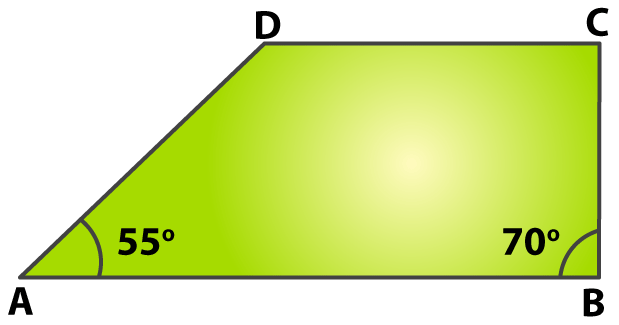Solution:

It is given that AB || DC

From the figure we know that ∠ A and ∠ D are consecutive interior angles

So it can written as

∠ A + ∠ D = 180o

To find ∠ D

∠ D = 180o – ∠ A

By substituting the value in the above equation

∠ D = 180o – 55o

By subtraction

∠ D = 125o

In a quadrilateral we know that the sum of all the angles is 360o

So we can write it as

∠ A + ∠ B + ∠ C + ∠ D = 360o

By substituting the values in the above equation

55o + 70o + ∠ C + 125o = 360o

On further calculation

∠ C = 360o – 55o – 70o – 125o

By subtraction

∠ C = 360o – 250o

∠ C = 110o

Therefore, ∠ C = 110o and ∠ D = 125o.

4. In the adjoining figure, ABCD is a square and △ EDC is an equilateral triangle. Prove that

(i) AE = BE,

(ii) ∠ DAE = 15o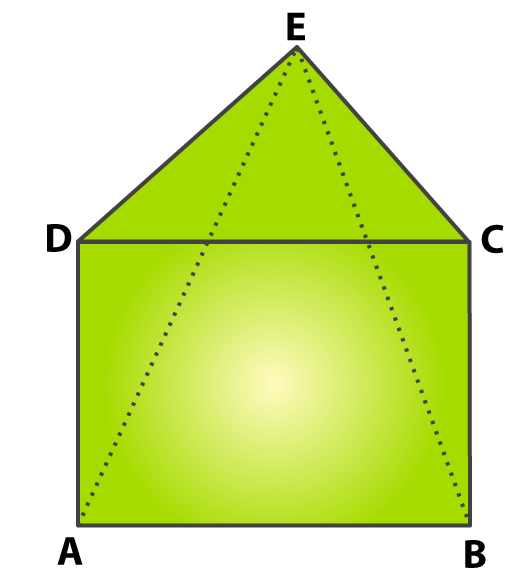Solution:

(i) From the figure we know that △ EDC is an equilateral triangle.

So we get ∠ EDC = ∠ ECD = 60o

We know that ABCD is a square

So we get ∠ CDA = ∠ DCB = 90o

Consider △ EDA

We get

∠ EDA = ∠ EDC + ∠ CDA

By substituting the values in the above equation

∠ EDA = 60o + 90o

So we get

∠ EDA = 150o ……. (1)

Consider △ ECB

We get

∠ ECB = ∠ ECD + ∠ DCB

By substituting the values in the above equation

∠ ECB = 60o + 90o

So we get

∠ ECB = 150o

So we get ∠ EDA = ∠ ECB …… (2)

Consider △ EDA and △ ECB

From the figure we know that ED and EC are the sides of equilateral triangle

So we get

ED = EC

We also know that the sides of square are equal

DA = CB

By SAS congruence criterion

△ EDA ≅ △ ECB

AE = BE (c. p. c. t)

(ii) Consider △ EDA

We know that

ED = DA

From the figure we know that the base angles are equal

∠ DEA = ∠ DAE

Based on equation (1) we get ∠ EDA = 150o

By angle sum property

∠ EDA + ∠ DAE + ∠ DEA = 180o

By substituting the values we get

150o + ∠ DAE + ∠ DEA = 180o

We know that ∠ DEA = ∠ DAE

So we get

150o + ∠ DAE + ∠ DAE = 180o

On further calculation

2 ∠ DAE = 180o – 150o

By subtraction

2 ∠ DAE = 30o

By division

∠ DAE = 15o

5. In the adjoining figure, BM ⊥ AC and DN ⊥ AC. If BM = DN, prove that AC bisects BD.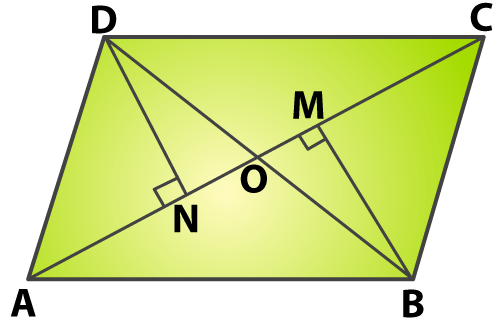Solution:

It is given that BM ⊥ AC and DN ⊥ AC

From the figure we know that ∠ DON and ∠ MOB are vertically opposite angles

∠ DON = ∠ MOB

Since DN and MB are perpendiculars

We know that

∠ DNO = ∠ BMO = 90o

It is given that BM = DN

By AAS congruence criterion

△ DNO ≅ △ BMO

OD = OB (c. p. c. t)

Therefore, it is proved that AC bisects BD.

6. In the given figure, ABCD is a quadrilateral in which AB = AD and BC = DC. Prove that

(i) AC bisects ∠ A and ∠ C,

(ii) BE = DE,

(iii) ∠ ABC = ∠ ADC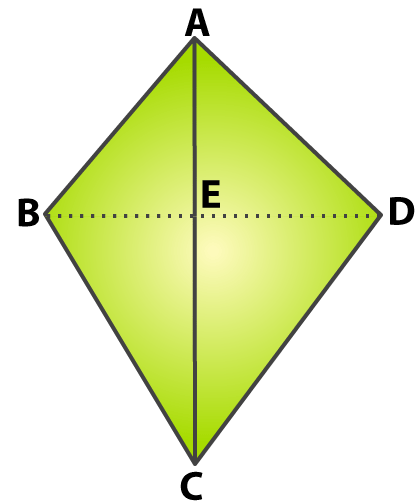Solution:

(i) Consider △ ABC and △ ADC

It is given that AB = AD and BC = DC

AC is common i.e. AC = AC

By SSS congruence criterion

△ ABC ≅ △ ADC ……… (1)

∠ BAC = ∠ DAC (c. p. c. t)

So we get

∠ BAE = ∠ DAE

We know that AC bisects the ∠ BAD i.e. ∠ A

So we get

∠ BCA = ∠ DCA (c. p. c. t)

It can be written as

∠ BCE = ∠ DCE

So we know that AC bisects ∠ BCD i.e. ∠ C

(ii) Consider △ ABE and △ ADE

It is given that AB = AD

AE is common i.e. AE = AE

By SAS congruence criterion

BE = DE (c. p. c. t)

(iii) We know that △ ABC ≅ △ ADC

Therefore, by c. p. c. t ∠ ABC = ∠ ADC

7. In the given figure, ABCD is a square and ∠ PQR = 90o. If PB = QC = DR, prove that

(i) QB = RC,

(ii) PQ = QR,

(iii) ∠ QPR = 45o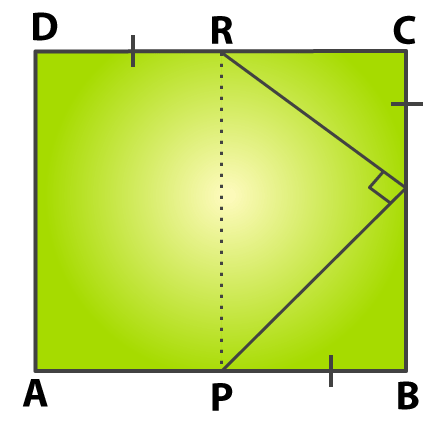Solution:

(i) We know that the line segment QB can be written as

QB = BC – QC

Since ABCD is a square we know that BC = DC and QC = DR

So we get

QB = CD – DR

From the figure we get

QB = RC

(ii) Consider △ PBQ and △ QCR

It is given that PB = QC

Since ABCD is a square we get

∠ PBQ = ∠ QCR = 90o

By SAS congruence criterion

△ PBQ ≅ △ QCR

PQ = QR (c. p. c. t)

(iii) We know that PQ = QR

Consider △ PQR

From the figure we know that ∠ QPR and ∠ QRP are base angles of isosceles triangle

∠ QPR = ∠ QRP

We know that the sum of all the angles in a triangle is 180o

∠ QPR + ∠ QRP + ∠ PRQ = 180o

By substituting the values in the above equation

∠ QPR + ∠ QRP + 90o = 180o

On further calculation

∠ QPR + ∠ QRP = 180o – 90o

By subtraction

∠ QPR + ∠ QRP = 90o

We know that ∠ QPR = ∠ QRP

So we get

∠ QPR + ∠ QPR = 90o

2 ∠ QPR = 90o

By division

∠ QPR = 45o

Therefore, it is proved that ∠ QPR = 45o.

8. If O is a point within a quadrilateral ABCD, show that OA + OB + OC + OD > AC + BD.

Solution:It is given that O is a point within a quadrilateral ABCD

Consider △ ACO

We know that in a triangle the sum of any two sides is greater than the third side

We get

OA + OC > AC ….. (1)

In the same way

Consider △ BOD

We get

OB + OD > BD ….. (2)

By adding both the equations we get

OA + OC + OB + OD > AC + BD

Therefore, it is proved that OA + OC + OB + OD > AC + BD.

9. In the adjoining figure, ABCD is a quadrilateral and AC is one of its diagonals. Prove that

(i) AB + BC + CD + DA > 2AC

(ii) AB + BC + CD > DA

(iii) AB + BC + CD + DA > AC + BD.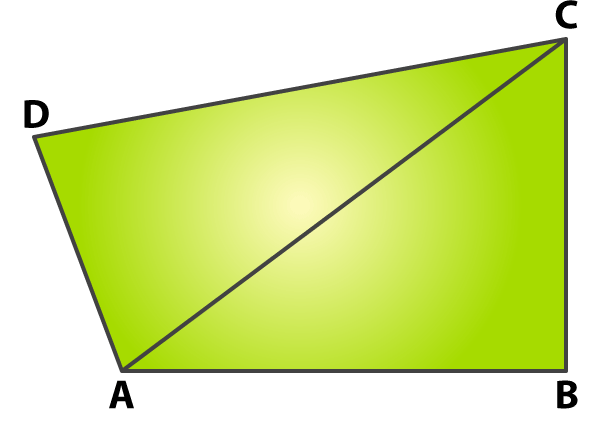Solution:

(i) Consider △ ABC

We know that

AB + BC > AC …… (1)

Consider △ ACD

We know that

AD + CD > AC ……. (2)

By adding both the equations we get

AB + BC + AD + CD > AC + AC

So we get

AB + BC + AD + CD > 2AC …….. (3)

Therefore, it is proved that AB + BC + AD + CD > 2AC.

(ii) Consider △ ABC

We know that

AB + BC > AC

Add CD both sides of the equation

AB + BC + CD > AC + CD …… (4)

Consider △ ACD

We know that

AC + CD > DA ……. (5)

By substituting (5) in (4) we get

AB + BC + CD > DA …….. (6)

(iii) Consider △ ABD and △ BDC

We know that

AB + DA > BD ……. (7)

So we get

BC + CD > BD ……. (8)

By adding (7) and (8) we get

AB + DA + BC + CD > BD + BD

On further calculation

AB + DA + BC + CD > 2BD ……. (9)

By adding equations (9) and (3) we get

AB + DA + BC + CD + AB + BC + AD + CD > 2BD + 2AC

So we get

2 (AB + BC + CD + DA) > 2 (BD + AC)

Dividing by 2

AB + BC + CD + DA > BD + AC

Therefore, it is proved that AB + BC + CD + DA > BD + AC.

10. Prove that the sum of all the angles of a quadrilateral is 360o.

Solution: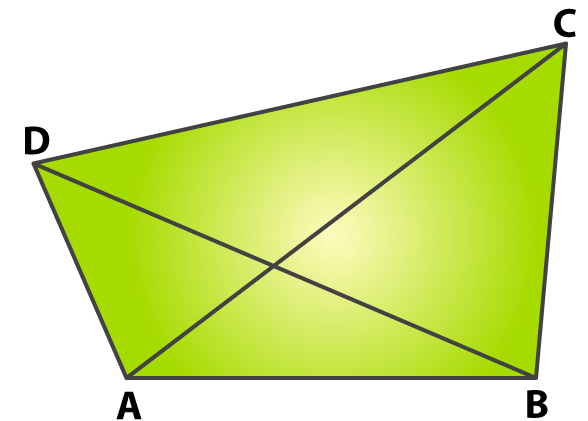Consider △ ABC

We can write it as

∠ CAB + ∠ B + ∠ BCA = 180o …….. (1)

Consider △ ACD

We can write it as

∠ DAC + ∠ ACD + ∠ D = 180o ……… (2)

Adding equations (1) and (2) we get

∠ CAB + ∠ B + ∠ BCA + ∠ DAC + ∠ ACD + ∠ D = 180o + 180o

So we get

∠ CAB + ∠ DAC + ∠ B + ∠ BCA + ∠ ACD + ∠ D = 360o

So it can be written as

∠ A + ∠ B + ∠ C + ∠ D = 360o

### Access other exercise solutions of Class 9 Maths Chapter 10: Quadrilaterals

Exercise 10B Solutions 27 Questions

Exercise 10C Solutions 18 Questions

### RS Aggarwal Solutions Class 9 Maths Chapter 10 – Quadrilaterals Exercise 10A

RS Aggarwal Solutions Class 9 Maths Chapter 10 Quadrilaterals Exercise 10A explains in brief about the types of quadrilaterals and various methods which are used in solving the exercise problems.

### Key features of RS Aggarwal Solutions for Class 9 Maths Chapter 10: Quadrilaterals Exercise 10A

• The solutions are prepared by experts in Mathematics in order to improve logical thinking among students.
• Diagramatic presentation of the questions help students to understand the concepts in a shorter period.
• The main aim is to make students knowledge driven and help them perform better in the exams.
• The faculty explains the concepts in an organized way based on the latest CBSE syllabus.